# Om Prakash

•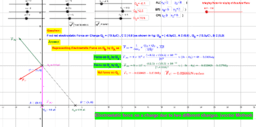### Electrostatic Force

Activity

Om Prakash

•### Electrostatic Force

Activity

Om Prakash

•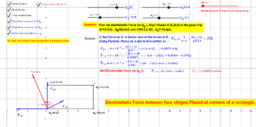### Electrostatic force between four charges.

Activity

Om Prakash

•### Electro Static Force Between Three Chages

Activity

Om Prakash

•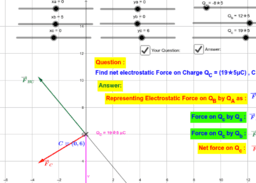### Electrostatic Force between three charges

Activity

Om Prakash

•### Electrostatic Force between three charges

Activity

Om Prakash

•### Variation in 'g' when going below the earth surface

Activity

Om Prakash

•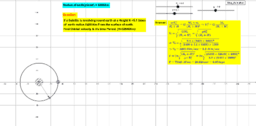### Orbital Velocity & it Time Period calculation

Activity

Om Prakash

•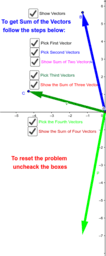### 3D demonstration to find out vector sum of 4 vectors

Activity

Om Prakash

•### Method of getting Vector Sum of Three Vector

Activity

Om Prakash

•### Vector double & Triple product

Activity

Om Prakash

•### Vector double & Triple product

Activity

Om Prakash

•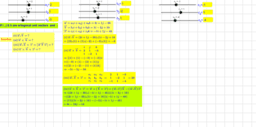### Vector Multiplication

Activity

Om Prakash

•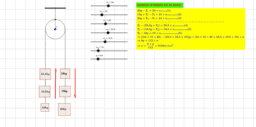### Use of Equation of Motion in pullies

Activity

Om Prakash

•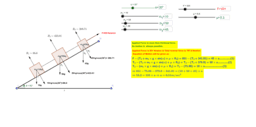### Motion on inclined plane (Rough/Smooth)

Activity

Om Prakash

•### Principle of Wheat Stone Bridge.

Activity

Om Prakash

•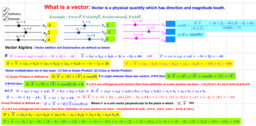### Vector Algebra

Book

Om Prakash

•### Vector Algebra

Activity

Om Prakash

•### Delta To Star To Delta Conversion Of Resistance Combination

Activity

Om Prakash

•### Delta to Star To Delta conversion of Capacitance combination

Activity

Om Prakash

•### Numerical Explanation of Star to Delta to star Conversion of Capacitance Combination

Activity

Om Prakash

•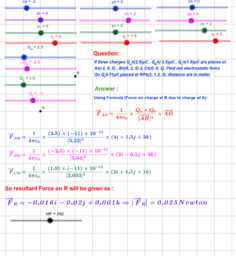### Electrostatic Force due to three charges at a single charge.

Activity

Om Prakash

•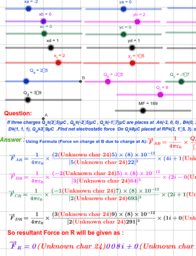### Electrostatic Force due to Four charges at a single Charge

Activity

Om Prakash

•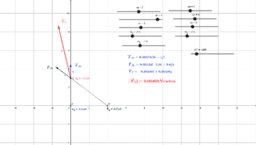Activity

Om Prakash